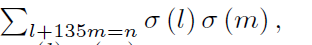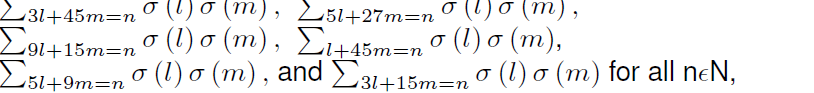##### Simultaneous Remotality in Operator Spaces

R. Khalil, S. Sammour

Journal of Advances in Mathematics and Computer Science, Page 451-456
DOI: 10.9734/BJMCS/2015/15521

The aim of this paper is to study simultaneous remotality in the Banach space of bounded linear operators on a Banach space H.

##### Eccentric Connectivity Index and Polynomial of Some Graphs

A. R. Bindusree, V. Lokesha, P. S. Ranjini

Journal of Advances in Mathematics and Computer Science, Page 457-463
DOI: 10.9734/BJMCS/2015/15137

Aims/ Objectives: Let G be a simple and connected graph with n vertices and m edges. The Eccentric connectivity index of G is defined as the summation of the product of degree and eccentricity of the vertices . Eccentric connectivity polynomial is a topological polynomial of G which is related to its Eccentric connectivity index . The objective of the study is to derive exact expressions of these indices for the double graph and extended double cover graph of a given graph. In addition to it, a lower bound of these invariants for the subdivision graph of the double graph and extended double cover graph of a given graph is also proposed.

Methodology: In this study, simple and connected graphs with n vertices and m edges are considered.
Results: In this article, exact expressions of Eccentric Connectivity index and Eccentric Connectivity Polynomial for the double graph of a given graph is presented. In addition to it, a lower bound of these invariants for the subdivision graph of the double graph of a given graph and a lower bound of these invariants for the extended double cover graph of a given graph is also proposed.

Conclusion: Eccentric Connectivity index and Eccentric Connectivity Polynomial of double graph and Extended double cover graphs can be expressed in terms of their parent graphs.

##### Adams Type Hybrid Block Methods Associated with Chebyshev Polynomial for the Solution of Ordinary Differential Equations

A. M. Badmus, Y. A. Yahaya, Y. C. Pam

Journal of Advances in Mathematics and Computer Science, Page 464-474
DOI: 10.9734/BJMCS/2015/14945

The new Hybrid Adams type Block Methods (HATBMs) for step length k=2,3 and 4 were developed for the solution of first order ordinary differential equations. Collocation and interpolation of Chebyshev polynomial approximation were adopted to derive some implicit linear multi-step methods at different values of k. Analysis of all the methods show that they were consistent, zero stable and convergent. All the newly constructed methods were demonstrated with numerical experiments to ascertain their level of convergence.

##### On Modelling of Promiscuous Lifestyle; An SIR Dynamics

W. Obeng-Denteh, B. A. Obeng, G. O. Fosu

Journal of Advances in Mathematics and Computer Science, Page 475-480
DOI: 10.9734/10.9734/BJMCS/2015/11815

An epidemiological model depicting the dynamics of campus sex among students is analyzed in this study. For the purpose of this research, sexual activities among students is considered as a disease called SESEX. The Susceptible - Infected - Recovered (SIR) model is used in analyzing the dynamics in this scenario. Two equilibrium points were found, a disease-free equilibrium and an endemic equilibrium point. The model suggests that, admitting new students without the infection plays a significant role in the reduction of SESEX on campus. Thus, minimizing recruitment of infected students reduces the spread of the disease.

##### The ε-Hyperstability of an Euler-Lagrange Type Quadratic Functional Equation in Banach Spaces

Mohamed Sirouni, Samir Kabbaj

Journal of Advances in Mathematics and Computer Science, Page 481-493
DOI: 10.9734/BJMCS/2015/14474

The main goal of this paper is the investigation of the ε-hyperstability of an Euler-Lagrange type quadratic functional equation

f(x + y) +1/2[f(x - y) + f(y - x)] = 2f(y) + 2f(x)

in the class of functions from an abelian group into a Banach space.

##### Evaluation of Some Convolution Sums and Representation Numbers of Quadratic Forms of Discriminant -135

Barış Kendirli

Journal of Advances in Mathematics and Computer Science, Page 494-531
DOI: 10.9734/BJMCS/2015/13973

We evaluate the convolution sumsusing the theory of quasimodular forms and determine the number of representations of a positive integer n by some direct sum of the formsof discriminant -135 by

modular forms. Moreover, we explain how to determine the number of representations of Q + tP, t > 1, t|135 for various sums Q , P of the above quadratic forms.

##### An Optimization Problem with Controls in the Coefficients of Parabolic Equations

M. H. Farag, T. A. Nofal, Mohamed A. El-Sayed, T. S. M. AL-Qarni

Journal of Advances in Mathematics and Computer Science, Page 532-543
DOI: 10.9734/BJMCS/2015/14738

In this paper, an optimal control parabolic problem is studied. The existence and uniqueness theorem for the solving optimal control parabolic problem is proved. Also, a theorem for the sufficient differentiability conditions of the functional and its gradient formulae has been proved.